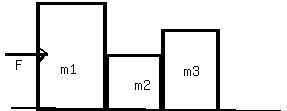Ask question

# The base of a triangle is 3 ft more than the height. If the area is 14 ft², find the base and the height.# The base of a triangle is 3 ft more than the height. If the area is 14 ft², find the base and the height.

Question
Right triangles and trigonometryasked 2021-02-05
The base of a triangle is 3 ft more than the height. If the area is 14 ft², find the base and the height.

## Answers (1)2021-02-06
The area of a triangle with base b and height h is given by: $$\displaystyle{A}=\frac{{1}}{{2}}{b}{h}$$
We are given that b=h+3 so we have: $$\displaystyle{14}=\frac{{1}}{{2}}{\left({h}+{3}\right)}$$ have
Multiply both sides by 2: $$\displaystyle{28}={h}^{{2}}+{3}{h}$$
Subtract 28 from both sides: $$\displaystyle{0}={h}^{{2}}+{3}{h}-{28}$$
Factor the right side: $$\displaystyle{0}={\left({h}+{7}\right)}{\left({h}-{4}\right)}$$
By zero product property: h=-7,4
The height cannot be negative so we have: h=4ft
which follows that: b=4+3
b=7ft

### Relevant Questionsasked 2021-03-12
Aidan knows that the observation deck on the Vancouver Lookout is 130 m above the ground. He measures the angle between the ground and his line of sight to the observation deck as $$\displaystyle{77}^{\circ}$$. How far is Aidan from the base of the Lookout to the nearest metre?asked 2020-12-15
A rectangle with dimensions 21.6 × 12 has a right triangle with a base 9.6 and a height of 7.2 cut out of the rectangle. What is the area of the shaded region?asked 2021-02-13
A vertical cylinder of cross-sectional area $$\displaystyle{0.050}{m}^{{2}}$$ is fitted with a tight-fitting, frictionless pistionof mass 5.0 kg. If there are 3.0 mol of ideal gas in the cylinderat 500 K, determine the height "h" at which the postion will be inequilibrium.asked 2021-03-24
The figure shows 3 crates being pushed over a concrete floor by a horizontal force f of magnitude 440N. The masses of the cratesare $$\displaystyle{m}_{{1}}={30}$$ kg, $$\displaystyle{m}_{{2}}={10}$$ kg, and $$\displaystyle{m}_{{3}}={20}$$ kg.The coefficient of kineticfriction between the floor and each of the crates is 0.7. a) what is the magnitude $$\displaystyle{F}_{{{32}}}$$ of the force on crate 3 from crate 2? b) If the crates then slide onto a polished floor, where the coefficientof kinetic friction is less than 0.700, is magnitude PSKF_{32}ZSL more than,less than, or the same as it was when the coeffient was 0.700?asked 2021-02-14
Dayton Power and Light, Inc., has a power plant on the Miami Riverwhere the river is 800 ft wide. To lay a new cable from the plantto a location in the city 2 mi downstream on the opposite sidecosts $180 per foot across the river and$100 per foot along theland.
(a) Suppose that the cable goes from the plant to a point Q on theopposite side that is x ft from the point P directly opposite theplant. Write a function C(x) that gives the cost of laying thecable in terms of the distance x.
(b) Generate a table of values to determin if the least expensivelocation for point Q is less than 2000 ft or greater than 2000 ftfrom point P.asked 2020-11-08
The shaded sector above covers $$\displaystyle\frac{{1}}{{2}}$$ of the circle. If the radius of the circle above is 3 cm, what is the area of the sector in terms of ππ. A. $$\displaystyle{1.125}π{c}{m}^{{2}}$$
B. $$\displaystyle{14.13}π{c}{m}^{{2}}$$
C. $$\displaystyle{4.5}π{c}{m}^{{2}}$$
D. $$\displaystyle{2.25}π{c}{m}^{{2}}$$asked 2021-03-24
A person bending forward to lift a load "with his back" rather than"with his knees" can be injured by large forces exerted on themuscles and vertebrae. A person is bending over to lift a200-N object. The spine and upper body are represented as auniform horizontal rod of weight 350 N, pivoted at the base of thespine. The erector spinalis muscle, attached at a pointtwo-thirds of the way up the spine, maintains the position of theback. The angle between the spine and this muscle is 12degrees. Find the tension in the back muscle and thecompressional force in the spine.asked 2020-10-18
Use function notation to describe the way the second variable (DV) depends upon the first variable (IV). Determine the domain and range for each, determine if there is a positive, negative, or no relationship, and explain your answers.
A)IV: an acute angle V in a right triangle: DV: the area B of the triangle if the hypotenuse is a fixed length G.
B)IV: one leg P of a right triangle: DV: the hypotenuse G of the right triangle if the other leg is 2
C)IV: the hypotenuse G of a right triangle: DV: the other leg P of the right triangle is one leg is 5.asked 2021-01-16
The length of the base of an isosceles triangle is x. The length of a leg is 2x - 6. The perimeter is 53. Find x.asked 2021-03-20
A cylindrical reservoir of diameter 4 feet and height 6 feet ishalf full of water weighing 62.5 lbs/ft3. The work done,in ft-lb, in emptying the water over the top is equal to $$\displaystyle\rho$$
...